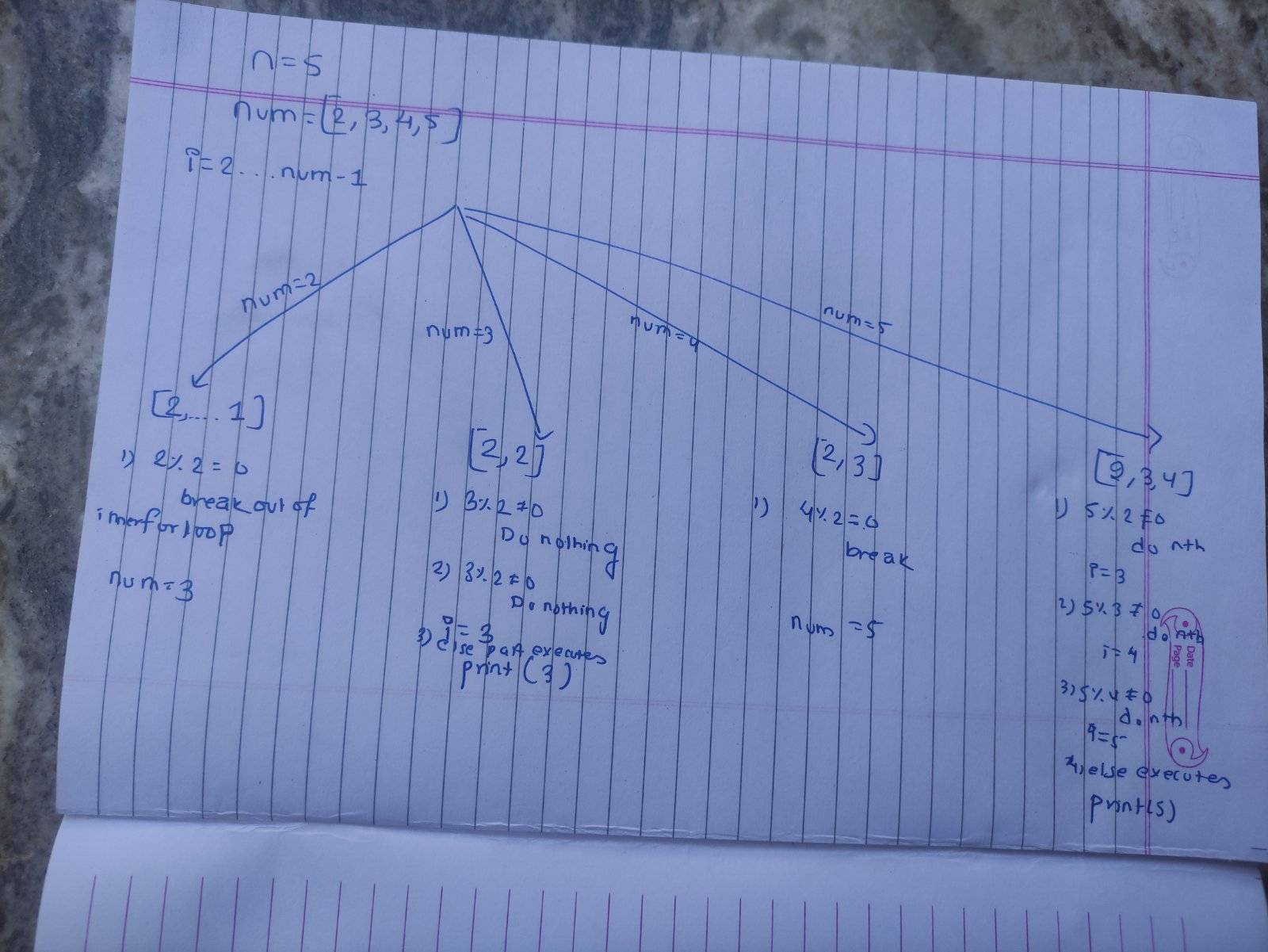# How does nested for loop works, can you explain with dry run?

• MHB
• shivajikobardan

#### shivajikobardan

Here is the code that I am talking about-:
Code:
n=int(input("Enter a number"))
for num in range(2,n+1):
for i in range(2,num):
if(num%i==0):
break
else:
print(num,end="")

If I give n=5 output should be 2,3,5.

Here is my dry run. Everything is fine except for 2 where I am not getting 2 as output. What has gone wrong here? I don't understand what is gone wrong here?Your else statement is not properly indented to align with the if statement. I believe it should be like this.

Code:
n=int(input("Enter a number"))
for num in range(2,n+1):
for i in range(2,num):
if(num%i==0):
break
else:
print(num,end="")

If n= 5 then the "outer loop" runs for num= 2 to num= 6.

For num= 2, the "inner loop" runs for i= 2 to i= 2 (in other words, i is only 2). 2 divided by 2 is 1 with no remainder so 2%2= 0 so "break" and go on to

For num= 3, the "inner loop runs for i= 2 to i= 3. 3 divided by 2 is 1 with remainder 1 so 3%2= 1 so print 3. 3 divided by 3 is 1 with no remainder so 3%3=0 so "break" and go on to

For num= 4, the inner loop runs for i= 2 to 4. 4 divided by 2 is 2 with no remainder so 2%2= 0 "break". 4 divided by 3 is 1 with remainder 1 so print 4. 4 divided by 4 is 1 with remainder 0 so 4%4= 0 "break"

For num= 5, the inner loop runs for i= 2 to 5. 5 divided by 2 is 2 with remainder 1 so 5%2= 1 so print 5. 5 divided by 3 is 1 with remainder 2 so 5%3= 2 so print 5. 5 divided by 4 is 1 with remainder 1 so 5%4= 1 so print 5. 5 divided by 5 is 1 with remainder 0 so break.

For num= 6, the inner loop runs for i= 2 to 6. 6 divided by 2 is 3 with no remainder so 6%2= 0 so break. 6 divided by 3 is 2 with no remainder so 6%3= 0 so break. 6 divided by 4 is 1 with remainder 2 so 6%4= 2 so print 6. 6 divided by 5 is 1 with remainder 1 so 6%5= 1 so print 6. 6 divided by 6 is 1 with no remainder so 6%6= 0 so break.

The result will be to print "3 4 5 5 5 6 6".